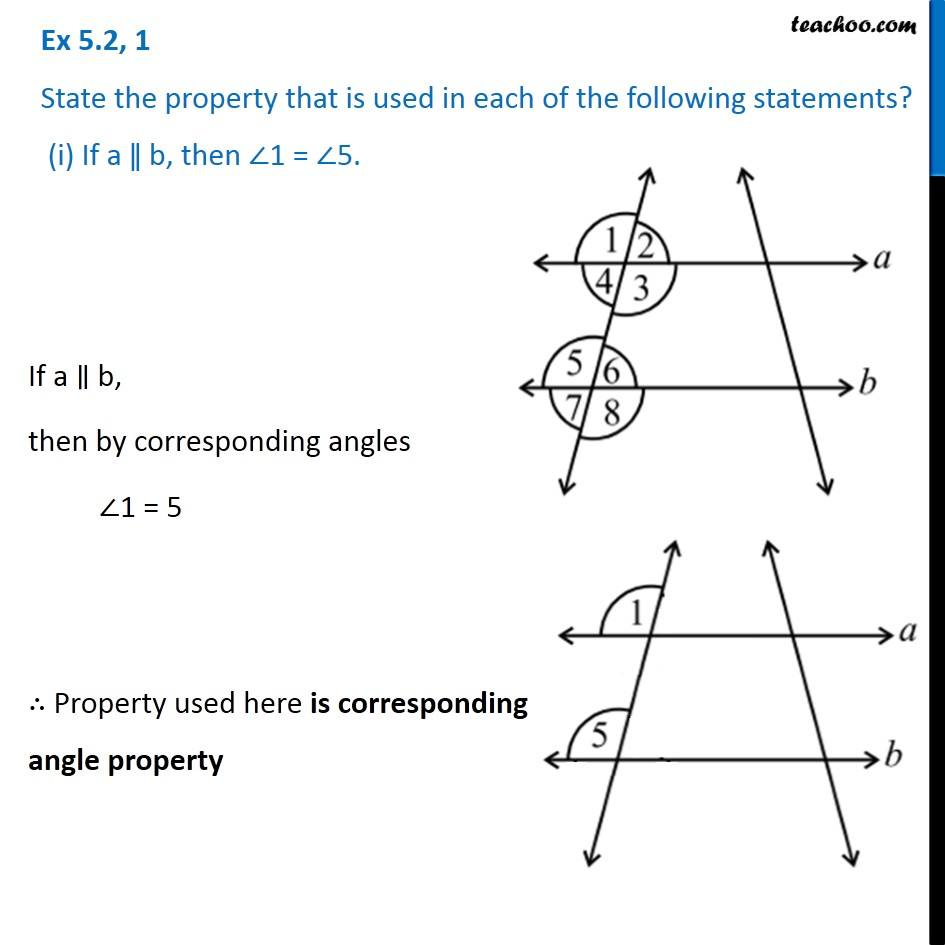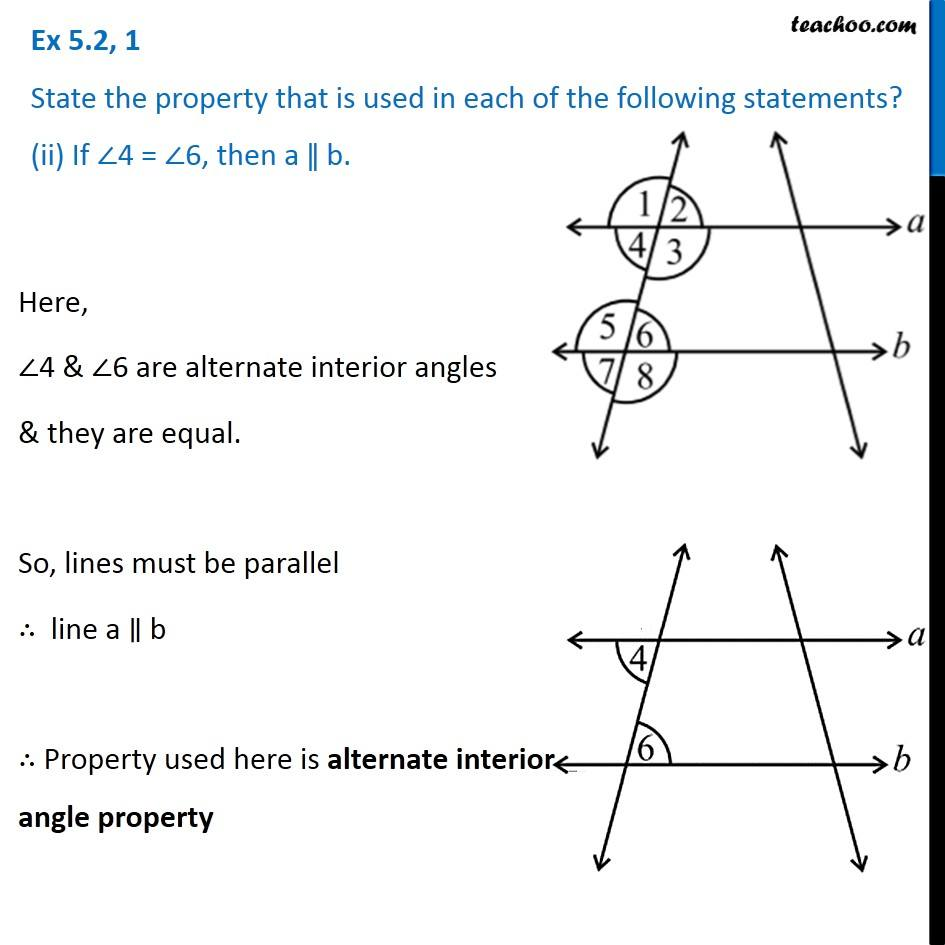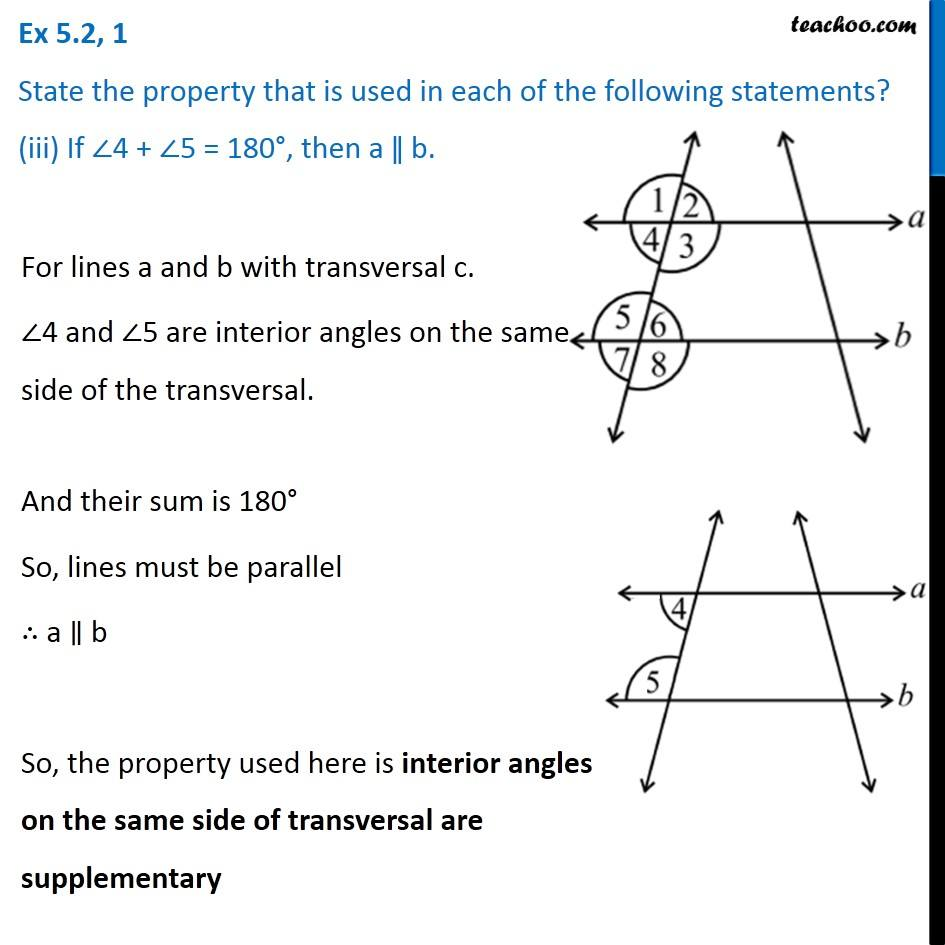Ex 5.2

Chapter 5 Class 7 Lines and Angles
Serial order wiseGet live Maths 1-on-1 Classs - Class 6 to 12

### Transcript

Ex 5.2, 1 State the property that is used in each of the following statements? (i) If a ǁ b, then ∠1 = ∠5. If a ∥ b, then by corresponding angles ∠1 = 5 ∴ Property used here is corresponding angle property If a ∥ b, then by corresponding angles ∠1 = 5 ∴ Property used here is corresponding angle property If a ∥ b, then by corresponding angles ∠1 = 5 ∴ Property used here is corresponding angle property Ex 5.2, 1 State the property that is used in each of the following statements? (ii) If ∠4 = ∠6, then a ǁ b. Here, ∠4 & ∠6 are alternate interior angles & they are equal. So, lines must be parallel ∴ line a ∥ b ∴ Property used here is alternate interior angle property Ex 5.2, 1 State the property that is used in each of the following statements? (iii) If ∠4 + ∠5 = 180°, then a ǁ b. For lines a and b with transversal c. ∠4 and ∠5 are interior angles on the same side of the transversal. And their sum is 180° So, lines must be parallel ∴ a ∥ b So, the property used here is interior angles on the same side of transversal are supplementary